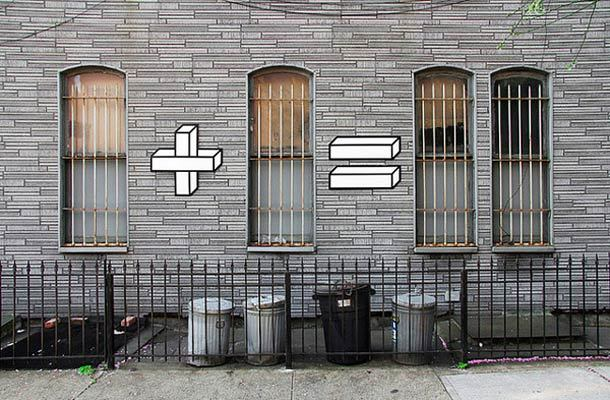# Top 30 Math puzzles everyone should tryMathematical puzzles or math puzzles are based on logics and a bit of mathematics. These are very important in development of logical mind. One with a habit of solving math puzzles develops logical thinking and considered a very high IQ person. These puzzles are regardless of age group as it requires only a bit of mathematics but high logics.

Math puzzles now a days is becoming interest of employers during interview processes, which allows employers to test IQ of the candidate. Here we’ve listed 30 most famous mathematical puzzles which must be tried by everyone of every age group. Once you are done with all the 30 puzzles, you are now well prepared for any interview puzzles.

Solve 2 puzzles from this list daily, and you are done! 🙂

List of 30 mathematical puzzles with answers, based on mathematics and out of the box logics(Don’t miss any, try all) –

### Defective ball out of 8 balls puzzle Burning Rope Problem Famous Handshake puzzle Blindfolded 10 Coins Puzzle Famous math equation puzzle Camel and banana puzzle Shopkeeper and fake note puzzle Ratio of Boys and Girls in a Country where people want only boys 5 liter measuring puzzle Marbles probability puzzle Greedy pirates and gold coin distribution Puzzle King and Wine Bottles Puzzle Crossing the Bridge Puzzle Random Airplane Seats puzzle 2 Eggs and 100 floor Google Classic question Measure 9 minutes from 2 hourglasses puzzle Sword Killing and Survival(Josephus problem) puzzle Defective chocolate box puzzle Red and Blue marbles Puzzle Gold Bar Problem 100 Doors Puzzle Bulbs in circle puzzle Sum and product confusion puzzle Biggest number using four 3s Puzzle 6 digits number forming puzzle Fingers multiplication trickey math puzzle Ant and Triangle Problem The Wall Street Investor puzzle Bank Cashier Puzzle Calandar cube puzzle

Done? Wow!! you are now a math puzzle champ 🙂